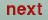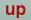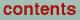Next: Fermi Surface plot Up: Plot programs Previous: Band structure plot

## Density of states plot

The program gnudos.run generates total or partial density of states plots. The program reads the file DOS created by lmdos.run and creates the plot data files DATA.DOS, FERMI.DOS and DOS.GNU. After the selection of the output device one is prompted for the energy units to be used:

` energies in Ryd.(f) or eV(t)? (default f)`
Entering t (logical true) selects electron volts, whereas f (logical false) or / selects Rydberg. The next prompt allows a shift of the energy origin to the Fermi energy:
`  energies relative to EF (t)? (default is f)`
Now the DOS file is read and information about it's content is displayed:
``` emin,emax= -1.0 .0, nopts=1001,
nd=24 efermi=-.1426

classes are: NA1  NA2  RB1  RB2  SB1  SB2
l's     are: s  p  d  f```
Next the projected DOS which should be added and plotted are selected:
``` Enter class1-l1 class2-l2 .. to be added to DOS
Examples:   all   s   p   NA1-f  SB2-s```
If `all' is entered, then the total density of states is plotted. Entering the name of one or several atoms yields a plot of the partial density of states associated with these atoms. Entering the orbital character (s,p,d,f) makes a-projected DOS which is summed over all atoms. If the name of a class of atoms augmented by `-' and an orbital character symbol is entered, only the partial density of states associated with these atoms and this specific-value is plotted. Additive combinations are also possible ( e.g. RB1 + s generates a plot of all s-orbitals present and all additional orbitals on the RB1 atoms).
Following a table of all selected orbitals, a choice of the energy range is prompted:
`Take: NA1-s NA1-p NA1-d NA2-s NA2-p NA2-df`
Following the table of all selected orbitals you are prompted for the relative weight of each partial DOS:
``` Now enter weights for partial DOS
(default=1,1,..)
A weight of 1.0 for each partial DOS gives
the correct total DOS.```
Entering / for the default yields the correct total DOS, but you may enter a number for each partial DOS. Next you are prompted for the energy range:
``` emin  , emax   =  -1.000    .000
if desired, enter new emin emax, / for default```
Entering / instead of two numbers selects the whole range shown above. The energy units are the ones selected in the first step. The default range is defined in the DOS category in the CTRL file. The next prompt permits changing the range of the density of states in exactly the same way:
``` dosmin, dosmax =.0 1070.419
if desired, enter new min max,/ for default
/
new values for emin, emax, dmin, dmax are:
-1.000       .000      .000     1080.0

title = all
if desired, enter new title / for default```
Finally a title for the plot can be given: Now the plotfile or the plot window should be generated.Next: Fermi Surface plot Up: Plot programs Previous: Band structure plot

O. Jepsen
Thu Oct 12 14:48:45 MESZ 2000